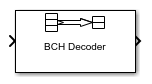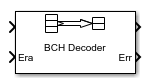# BCH Decoder

Decode BCH code to recover binary vector data

• Library:
• Communications Toolbox / Error Detection and Correction / Block## Description

The BCH Decoder block recovers a binary message vector from a binary BCH codeword vector. For proper decoding, the Codeword length, N and Message length, K parameter values in this block must match the parameters in the corresponding BCH Encoder block. The full-length values of N and K must produce a valid narrow-sense BCH code.

If the encoder is processing multiple codewords per frame, then the same puncture pattern holds for all codewords. The input and output signal lengths are listed in Input and Output Signal Length in BCH Blocks.

See Tips for information about valid N values, valid (N,K) pairs, and error-correcting capabilities for a given BCH code.

If decoding fails, the message portion of the decoder input is returned unchanged as the decoder output.

The sample times of all input and output signals are equal.

This icon shows optional ports.## Ports

### Input

expand all

Encoded message, specified as a binary column vector. The encoded message is a BCH code with message length K and codeword length (N – number of punctures).

Data Types: `single` | `double` | `int8` | `int16` | `int32` | `uint8` | `uint16` | `uint32` | `Boolean`

Erasure vector, specified as a binary column vector that is the same length as `In`. Erasure values of 1 correspond to erased bits in the same position in the codeword. Values of 0 correspond to bits that are not erased.

#### Dependencies

To enable this port, select Enable erasures input port.

Data Types: `double` | `Boolean`

### Output

expand all

Decoded message, returned as a binary column vector input signal with an integer multiple of Message length, K elements or Shortened message length, S elements if the code is shortened. Each group of input elements represents one codeword to decode. The input and output signal lengths are listed in the Input and Output Signal Length in BCH Blocks table.

Data Types: `single` | `double` | `int8` | `int16` | `int32` | `uint8` | `uint16` | `uint32` | `Boolean`

Decoding errors, returned as an integer vector that indicates the number of errors detected during decoding of the codeword. A negative integer indicates that the block detected more errors than it could correct by using the coding scheme.

#### Dependencies

To enable this port, select Output number of corrected errors.

Data Types: `single` | `double` | `int8` | `int16` | `int32` | `uint8` | `uint16` | `uint32` | `Boolean`

## Parameters

expand all

Codeword length, specified as an integer of the form N = 2M–1, where M is an integer from 3 through 16. For more information, see Tips.

Message length, specified as an integer. The (`N`, `K`) pair must produce a narrow-sense BCH code.

Shortened message length, specified as an integer. When you specify this parameter, provide full-length N and K values to specify the (N, K) code that is shortened to an (NK+S, S) code.

#### Dependencies

To enable this parameter, select .

Generator polynomial, specified as one of the following:

• A polynomial character vector — For more information, see Character Representation of Polynomials.

• A binary row vector that represents the coefficients of the generator polynomial in order of descending power.

• A binary Galois row vector that represents the coefficients of the generator polynomial in order of descending power.

Example: `'X^10 + X^8 + X^5 + X^4 + X^2 + X + 1'`, which is equivalent to `bchgenpoly(15,5)`

#### Dependencies

To enable this parameter, select .

Primitive polynomial in order of descending power. It is a polynomial of order M that defines the finite Galois field GF(2), specified as one of the following:

• A polynomial character vector — For more information, see Character Representation of Polynomials.

• A binary row vector that represents the coefficients of the generator polynomial in order of descending power.

Example: `'X^4 + X + 1'`, which is the primitive polynomial used for a (15,5) code, `de2bi(primpoly(4,'nodisplay'),'left-msb')`

#### Dependencies

To enable this parameter, select .

Select this parameter to disable generator polynomial check.

Each time a model initializes, the block performs a polynomial check. This check verifies that X N + 1 is divisible by the specified generator polynomial, where N represents the full codeword length. For larger codes, disabling the check speeds up the simulation process.

### Tip

Always run the check at least once before disabling this feature.

#### Dependencies

To enable this parameter, select .

Puncture vector, specified as a binary column vector of length NK. Element indices with `1`s represent data symbol indices that pass through the block unaltered. Element indices with `0`s represent data symbol indices that get punctured, or removed, from the data stream. For more information, see Puncturing and Erasures.

#### Dependencies

To enable this parameter, select .

Selecting this check box enables the erasures port, `Era`.

Through the port, you can input a binary column vector that is 1/M times as long as the codeword input.

Erasure values of `1` correspond to erased symbols in the same position in the bit-packed codeword. Values of `0` correspond to nonerased symbols. For more information, see Puncturing and Erasures.

Selecting this check box enables an additional output port, `Err`, which indicates the number of errors the block corrected in the input codeword.

## Block Characteristics

 Data Types `Boolean` | `double` | `integer` | `single` Multidimensional Signals `no` Variable-Size Signals `no`

expand all

## Tips

• To generate the list of valid (`N`,`K`) pairs along with the corresponding values of the error-correction capability, run `bchnumerr`(`N`).

• Valid values for `N` = 2M–1, where M is an integer from 3 through 16. The maximum allowable value of `N` is 65,535.

## Algorithms

This block implements the algorithm, inputs, and outputs described in Algorithms for BCH and RS Errors-only Decoding.

 Wicker, Stephen B. Error Control Systems for Digital Communication and Storage. Upper Saddle River, NJ: Prentice Hall, 1995.

 Berlekamp, Elwyn R. Algebraic Coding Theory. New York: McGraw-Hill, 1968.

 Clark, George C., Jr., and J. Bibb Cain. Error-Correction Coding for Digital Communications. New York: Plenum Press, 1981.